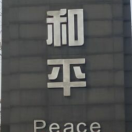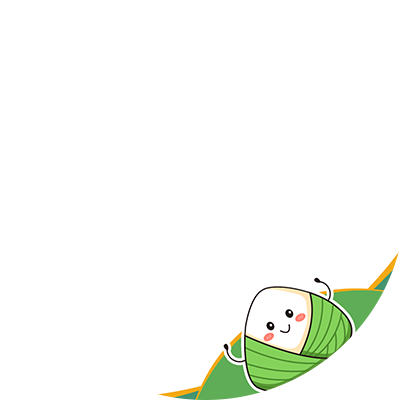# 五分钟拿捏 Python 字典 -Python3 入门必备 [字典详细操作]

• 2022 年 7 月 14 日
• 本文字数：2488 字

阅读完需：约 8 分钟

### 介绍

d = {key1 : value1, key2 : value2, key3 : value3 }

1.字典中的键必须是唯一的，而且，虽然键不指定键的数据类型，但是键只能使用不可变对象作为键（比如：字符串）。

2.dict 作为 Python 的关键字和内置函数，变量名不建议命名为 dict。

### 字典的使用

ele = {'H':'Hydrogen','He':'Helium','Li':'Lithium','Be':'Beryllium','C':'Carbon','C':'23'}print(ele)#打印结果:{'H': 'Hydrogen', 'He': 'Helium', 'Li': 'Lithium', 'Be': 'Beryllium', 'C': '23'}

#### 字典的创建

##### 通过指定键值创建字典

d = dict.fromkeys(['a','b'], 12) #创建一个键分别为a,b;值为12的字典print(d) #打印结果: {'a': 12, 'b': 12}

zd = dict(zip(keys, vals))#打印结果:{'a': 1, 'b': 2} 有点类似fromkeys()只不过后面的值是一个列表或者元组,可分别对应赋值

##### 直接使用 dict()创建
D = dict(a='123', b='hello')

##### 通过 for 循环创建一个字典
d3 = {k:k*2 for k in range(4)}print(d3)#结果:{0: 0, 1: 2, 2: 4, 3: 6}

#### 读取字典

##### 返回对应键的值
d = dict(a='123', b='hello')print(d['a'])#打印结果:123

d = dict(a='123', b='hello')print(d.get('b'))#结果:hello

##### 判断键是否在字典中

print('a' in d) #True

##### 以列表的形式返回字典的键和值

print(d.keys()) #结果:dict_keys(['a', 'b'])print(d.values()) #结果:dict_values(['123', 'hello'])

print(list(d.keys())) #结果:['a', 'b']

##### 以列表的形式返回字典中可遍历的元素

d = dict(a='123', b='hello',c=[1,2,3])print(d.items())#结果:dict_items([('a', '123'), ('b', 'hello'), ('c', [1, 2, 3])])

##### 字典的复制

d = dict(a='123', b='hello',c=[1,2,3])print(d.copy())print(d)#结果如下:#复制的字典:{'a': '123', 'b': 'hello', 'c': [1, 2, 3]}#原字典:{'a': '123', 'b': 'hello', 'c': [1, 2, 3]}

##### 获取字典的元素个数
d = dict(a='123', b='hello',c=[1,2,3])print(len(d))#结果:3

#### 字典添加元素

d = dict(a='123', b='hello',c=[1,2,3]) #{'a': '123', 'b': 'hello', 'c': [1, 2, 3]}d3 = {k:k*2 for k in range(4)} #{0: 0, 1: 2, 2: 4, 3: 6}d.update(d3)print(d)#结果:{'a': '123', 'b': 'hello', 'c': [1, 2, 3], 0: 0, 1: 2, 2: 4, 3: 6}

#### 字典的修改元素

d = dict(a='123', b='hello',c=[1,2,3]) #{'a': '123', 'b': 'hello', 'c': [1, 2, 3]}d['c'] = '更新后的值'print(d) #{'a': '123', 'b': 'hello', 'c': '更新后的值'}

#### 字典的删除元素

d = dict(a='123', b='hello',c=[1,2,3]) #{'a': '123', 'b': 'hello', 'c': [1, 2, 3]}print(d.pop('a'))#结果:123print(d) #{'b': 'hello', 'c': [1, 2, 3]}

d = dict(a='123', b='hello',c=[1,2,3]) #{'a': '123', 'b': 'hello', 'c': [1, 2, 3]}del d['a']print(d) #{'b': 'hello', 'c': [1, 2, 3]}

### 总结

1. 字典是一种映射机制,它不是序列,也没有顺序的概念,所以不要对字典使用类似字符串和序列的那种切片操作

2. 键不一定总是字符串,其实任何不可变对象都可以作为字典的键,例如:整数## 评论